## Projection methods in linear algebra numerics

Linear algebra classes often jump straight to the definition of a projector (as a matrix) when talking about orthogonal projections in linear spaces. As often as it happens, it is not clear how that definition arises. This is what is covered in this post.

## Orthogonal projection: how to build a projector

### Case 1 – 2D projection over (1,0)

It is quite straightforward to understand that orthogonal projection over (1,0) can be practically achieved by zeroing out the second component of any 2D vector, at last if the vector is expressed with respect to the canonical basis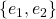. Albeit an idiotic statement, it is worth restating: the orthogonal projection of a 2D vector amounts to its first component alone.

How can this be put math-wise? Since we know that the dot product evaluates the similarity between two vectors, we can use that to extract the first component of a vector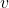. Once we have the magnitude of the first component, we only need to multiply that by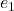itself, to know how much in the direction ofwe need to go. For example, starting from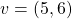, first we get the first component as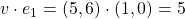; then we multiply this value by e_1 itself: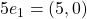. This is in fact the orthogonal projection of the original vector. Writing down the operations we did in sequence, with proper transposing, we get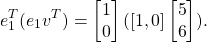One simple and yet useful fact is that when we project a vector, its norm must not increase. This should be intuitive: the projection process either takes information away from a vector (as in the case above), or rephrases what is already there. In any way, it certainly does not add any. We may rephrase our opening fact with the following proposition:

PROP 1: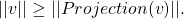This is can easily be seen through the pitagorean theorem (and in fact only holds for orthogonal projection, not oblique):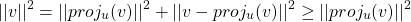## Reproducing a transport instability in convection-diffusion equation

Drawing from Larson-Bengzon FEM book, I wanted to experiment with transport instabilities. It looks there might be an instability in my ocean-ice model but before being able to address that, I wanted to wrap my head around the 1D simplest example one could find. And that is the Convection-Diffusion Equation:

(1)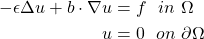The first term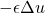is responsible of the smearing of the velocity field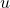proportionally to, and is thus called the diffusion term. Intuitively, it controls how much the neighbors of a given pointare influenced by the behavior of; how much velocities (or temperatures: think of whatever you want!) diffuse in the domain.

The second term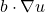controls how much the velocitiesare transported in the direction of the vector field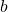, and is thus called the convective term. A requirement for this problem to be well-posed is that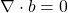— otherwise it would mean that we allow velocities to vanish or to come into existence.

The instability is particularly likely to happen close to a boundary where a Dirichlet boundary condition is enforced. The problem is not localized only close to the boundary though, as fluctuations can travel throughout the whole domain and lead the whole solution astray.

## Transport instability in 1D

The simplest convection-diffusion equation in 1D has the following form:

(2)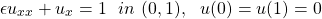whose solution, for smallis approximately just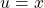. This goes well with the boundary condition at 0, but not with the condition at 1, where the solution needs to go down to 0 quite abruptly to satisfy the boundary condition.

It’s easy to simulate the scenario with FEniCS and get this result (with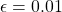and the unit interval divided in 10 nodes):in which we can infer two different trends: one with odd points and one with even points! In fact, if we discretize the 1D equation with finite elements we obtain:

(3)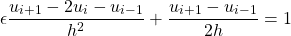## What is the Rossby number?

The Rossby number is used to describe whether a phenomenon is large-scale, i.e. if it is affected by earth’s rotation. But do we actually quantify if a fluid flow is affected by earth’s rotation?

Consider two quantities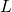and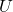, withbeing a characteristic scale-length of the phenomenon (ex. distance between two peaks, distance between two isobars, length of simulation domain) andthe horizontal velocity scale of the motion. The ratio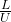is the time it takes to the motion to cover a distancewith velocity. If this time is bigger than the period of earth’s rotation, then the phenomenon IS affected by the rotation.

So if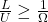, then the phenomenon IS a large-scale one. Thus we can define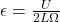and say that for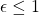a phenomenon is large scale. Phenomena with small Rossby number are dominated by Coriolis force behavior, while those with large Rossby number are dominated by inertial forces (ex: a tornado). However, rotational effects are more evident for low latitudes (i.e. near the equator), so the Rossby number can be different depending on where on earth we are.

(Notice that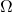is in theory equal to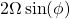, withbeing the earth rotational velocity and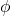the angle between the axis of rotation and the direction of fluid movement. In the geophysical context, flows are mostly horizontal (also due to density stratification in both atmosphere and ocean), so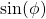can be approximated with 1. There is a bunch of different notation, but thisis also referred to as, called the Coriolis frequency.)

## How do Dirichlet and Neumann boundary conditions affect Finite Element Methods variational formulations?

To solve a classical second-order differential problem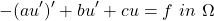with FEM, we first need to derive its weak formulation. This is achieved by multiplying the equation by a test functionand then integrating by parts to get rid of second order derivatives:

(1)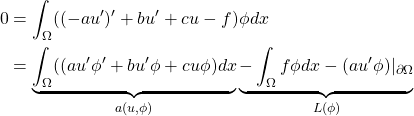A typical FEM problem then reads like: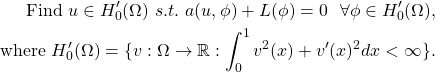What is the difference between imposing Dirichlet boundary conditions (ex.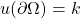) and Neumann ones (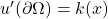) from a math perspective? Dirichlet conditions go into the definition of the space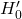, while Neumann conditions do not. Neumann conditions only affect the variational problem formulation straight away.

## A gentle (and short) introduction to Gröbner Bases

Taken from my report for a Computer Algebra course.

## Motivation

We know there are plenty of methods to solve a system of linear equations (to name a few: Gauss elimination, QR or LU factorization). In fact, it is straightforward to check whether a linear system has any solutions, and if it does, how many of them there are. But what if the system is made of non-linear equations? The invention of Groebner bases and the field of computational algebra came up to answer these questions.

In this text we will recap the theory behind single-variable polynomials and extend it to multiple-variable ones, ultimately getting to the definition of Groebner bases.

In some cases, the transition from one to multiple variables is smooth and pretty much an extension of the simple case (for example for the Greatest Common Divisor algorithm). In other cases, however, there are conceptual jumps to be made. To give an example, single variable polynomials have always a finite number of roots, while this does not hold for multivariable polynomials. Intuitively, the reason is that a polynomial in one variable describes a curve in the plane, which can only intersect the x-axis a discrete and finite number of times. On the other hand, a multivariate polynomial describes a surface in space, which will always intersect the 0-plane in a continuum of points.

## What is the difference between Finite Differences and Finite Element Methods?

With Finite Differences, we discretize space (i.e. we put  a grid on it) and we seek the values of the solution function at the mesh points. We still solve a discretized differential problem.

With Finite Elements, we approximate the solution as a (finite) sum of functions defined on the discretized space. These functions make up a basis of the space, and the most commonly used are the hat functions. We end up with a linear system whose unknowns are the weights associated with each of the basis functions: i.e., how much does each basis function count for out particular solution to our particular problem?

Brutally, it is finding the value of the solution function at grid points (finite differences) vs the weight of the linear combinations of the hat functions (finite elements).

## The role of intuitions in mathematics

Some thoughts and questions about the role of intuition in mathematics:

• Is intuition needed to really understand a topic?
I would say yes, since in the end we reason through ideas, of which we have an intuitive representation. Without intuitions, it is difficult to relate topics with each other as we lack in hooks, and we often lack a deep understanding as well.
• Do you feel like you have understood something even if you do not have an intuitive representation of it?
• How does formalism complete intuition?
It shows whether and how an intuition is right. Sometimes intuition can be deceitful and/or tricky, especially in high dimensions or very abstract topics.
• Can/Should intuitions be taught? Or are they only effective when discovered on one’s own?
I side more with the latter. This is bordering with Maths Education, but I deem the process more important than the result – it is the tough digestion of some math material that ultimately leads to developing an intuition what really makes the intuition strong in one’s mind. If somebody else (like a teacher) does the work for us, then the result does not really stick, albeit nice it may be.
• Can we say somebody with only intuitions (well understood and well reasoned) is a mathematician?
I would say yes. I often find the intuitive side more important than the formal one.
• Is it possible to develop intuitions for very abstract topics? If yes, what shape would they have, since there is rarely anything visual we can hook up to?

## A note on the hopes for Fully Homomorphic Signatures

This is taken from my Master Thesis on Homomorphic Signatures over Lattices.

## What are homomorphic signatures

Imagine that Alice owns a large data set, over which she would like to perform some computation. In a homomorphic signature scheme, Alice signs the data set with her secret key and uploads the signed data to an untrusted server. The server then performs the computation modeled by the function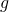to obtain the result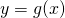over the signed data.

Alongside the result, the server also computes a signature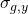certifying thatis the correct result for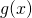. The signature should be short – at any rate, it must be independent of the size of. Using Alice’s public verification key, anybody can verify the tuplewithout having to retrieve all the data setnor to run the computationon their own again.

The signatureis a homomorphic signature, where homomorphic has the same meaning as the mathematical definition: ‘mapping of a mathematical structure into another one in such a way that the result obtained by applying the operations to elements of the first structure is mapped onto the result obtained by applying the corresponding operations to their respective images in the second one‘. In our case, the operations are represented by the function, and the mapping is from the matrices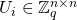to the matrices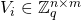.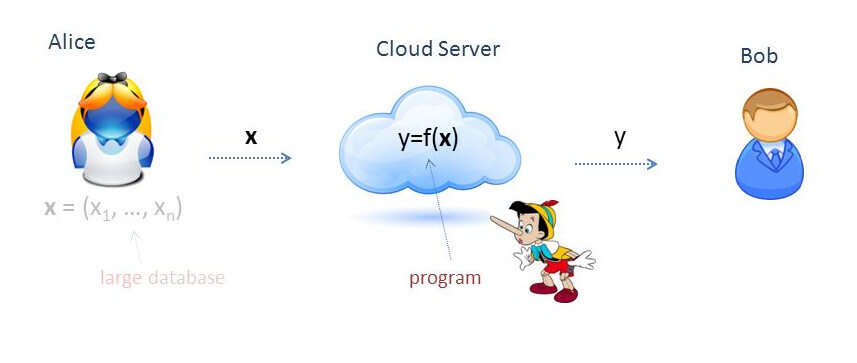Notice how the very idea of homomorphic signatures challenges the basic security requirements of traditional digital signatures. In fact, for a traditional signatures scheme we require that it should be computationally infeasible to generate a valid signature for a party without knowing that party’s private key. Here, we need to be able to generate a valid signature on some data (i.e. results of computation, like) without knowing the secret key. What we require, though, is that it must be computationally infeasible to forge a valid signature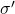for a result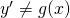. In other words, the security requirement is that it must not be possible to cheat on the signature of the result: if the provided result is validly signed, then it must be the correct result.

The next ideas stem from the analysis of the signature scheme devised by Gorbunov, Vaikuntanathan and Wichs. It relies on the Short Integer Solution hard problem on lattices. The scheme presents several limitations and possible improvements, but it is also the first homomorphic signature scheme able to evaluate arbitrary arithmetic circuits over signed data.

## Probability as a measure of ignorance

One of the most beautiful intuitions about probability measures came from Rovelli’s book, that took it in turn from Bruno de Finetti.

What does a probability measure measure? Sure, the open sets of the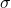-algebra that supports the measure space. But really, what? Thinking about it, it is very difficult to define probability without using the word probable or possible.

Well, probability measures our ignorance about something.

When we make some claim with 90% probability, what we are really saying is that the knowledge we have allows us to make a prediction that is that much accurate. And the main point here is that different people may assign different probabilities to the very same claim! If you have ever seen weather forecasts for the same day disagree, you know what I am talking about. Different data or different models can generate different knowledge, and thus different probability figures.

But we do not have to go that far to find reasonable examples. Let’s consider a very simple one. Imagine you found yourself on a train, and in front of you is sitting a girl with clothes branded Patagonia. What would be the odds that the girl has been to Patagonia? Not more than average, you would guess, because Patagonia is just a brand that makes warm clothes, and can be purchased in several stores all around the world, probably even more than in Patagonia itself! So you would probably say that is surely no more than 50% likely.

But now imagine a kid in the same scenario. If they see a girl with Patagonia clothes, they would immediately think that she had been to Patagonia (with probability 100% this time), because they are lacking a good amount of important information that you instead hold. And so the figure associated with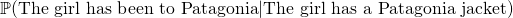is pretty different depending on the observer, or rather on the knowledge (or lack of) they possess. In this sense probability is a measure of our ignorance.

## But WHY is the Lattices Bounded Distance Decoding Problem difficult?

This is taken from my Master Thesis on Homomorphic Signatures over Lattices.

### Introduction to lattices and the Bounded Distance Decoding Problem

A lattice is a discrete subgroup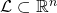, where the word discrete means that each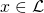has a neighborhood in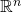that, when intersected with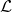results initself only. One can think of lattices as being grids, although the coordinates of the points need not be integer. Indeed, all lattices are isomorphic to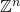, but it may be a grid of points with non-integer coordinates.Another very nice way to define a lattice is: givenindependent vectors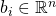, the latticegenerated by that base is the set of all linear combinations of them with integer coefficients: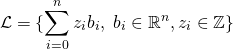Then, we can go on to define the Bounded Distance Decoding problem (BDD), which is used in lattice-based cryptography (more specifically, for example in trapdoor homomorphic encryption) and believed to be hard in general.

Given an arbitrary basis of a lattice, and a pointnot necessarily belonging to, find the point ofthat is closest to. We are also guaranteed thatis very close to one of the lattice points. Notice how we are relying on an arbitrary basis – if we claim to be able to solve the problem, we should be able to do so with any basis.

Now, as the literature goes, this is a problem that is hard in general, but easy if the basis is nice enough. So, for example for encryption, the idea is that we can encode our secret message as a lattice point, and then add to it some small noise (i.e. a small element). This basically generates an instance of the BDD problem, and then the decoding can only be done by someone who holds the good basis for the lattice, while those having a bad basis are going to have a hard time decrypting the ciphertext.